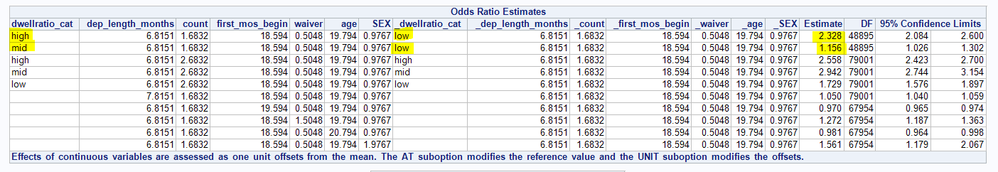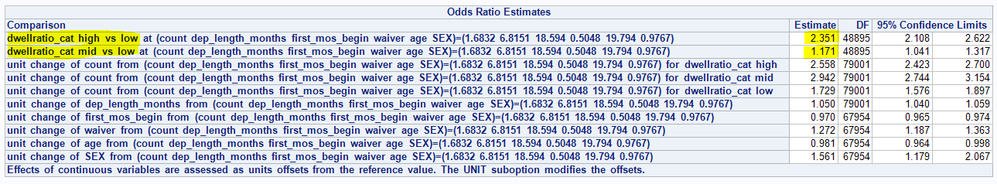## Multilevel logistic regression oddsratio statement

Can someone enlighten me as to why these different model statement specifications produce different results for some values?

```proc glimmix data=deployments_and_dwells ic=pq;
class randomid_adsaged dwellratio_cat(ref='low') officer2_begin marital_status_begin occupation_begin officer2_end marital_status_end occupation_end ;
model ptsd (event="1") = dwellratio_cat dep_length_months count dwellratio_cat*count first_mos_begin waiver age sex
/ dist=binary link=logit ddfm=bw solution cl oddsratio;
random intercept / subject = randomid_adsaged;
covtest / wald;
output out=nonmiss_data / nomiss;
where prior_ptsd = 0;
run;``````proc glimmix data=deployments_and_dwells ic=pq oddsratio;
class randomid_adsaged dwellratio_cat(ref='low') officer2_begin marital_status_begin occupation_begin officer2_end marital_status_end occupation_end ;
model ptsd (event="1") = dwellratio_cat dep_length_months count dwellratio_cat*count first_mos_begin waiver age sex
/ dist=binary link=logit ddfm=bw solution cl oddsratio(label at count=1.6832 at dep_length_months=6.8143 at first_mos_begin=18.588 at waiver=0.5048 at age=19.791 at sex=0.9767);
random intercept / subject = randomid_adsaged;
covtest / wald;
output out=nonmiss_data / nomiss;
where prior_ptsd = 0;
run;```## Re: Multilevel logistic regression oddsratio statement

In the first MODEL statement, the ODDSRATIO option evaluates the odds ratios where the continuous variables are set to their mean values.  In the second MODEL statement, the ODDSRATIO( at ... ) syntax evaluates the oddsratios at the specified values of the continuous variable. These are not the same values. For example, first_mos_begin=18.588 and age=19.791 are different values than in the first call.

Discussion stats